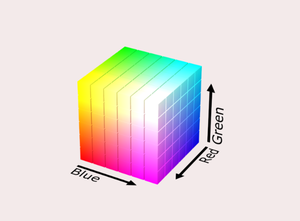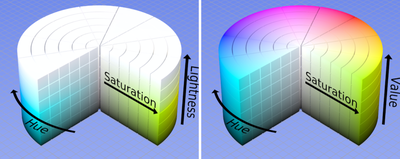## 前言

“色彩空间”一词源于西方的“Color Space”，又称作“色域”，色彩学中，人们建立了多种色彩模型，以一维、二维、三维甚至四维空间坐标来表示某一色彩，这种坐标系统所能定义的色彩范围即色彩空间。

## 常用的颜色模型

### RGB(A)颜色模型

• R：代表红色分量；
• G：代表绿色分量；
• B：代表蓝色分量；
• A：代表透明通道分量；### HSB颜色模型

HSB（也称HSV）颜色模型，是一种更符合直觉、更易于调色的颜色模型；

• HHue）：指色相，即单纯的颜色；取值范围为$[0,\ 360\degree]$
• SSaturation）：指饱和度，饱和度越高则颜色越纯；取值范围为$[0,\ 100\%]$
• BBrightness）：指明度，又称为值（Value）；取值范围为$[0,\ 100\%]$

HSB色彩空间为一个圆柱体：HSB色彩空间坐标实际上是一个极坐标系H分量为角度S分量为弧长B分量则是垂直于H-S极坐标系。

### HSB转RGB

\begin{aligned} h_i &= \lfloor \frac{h}{60} \rfloor \ mod\ 6 \\ f &= \frac{h}{60} - h_i \\ p &= v * (1 - s) \\ q &= v * (1 - f * s) \\ t &= v * (1 - (1 - f) * s) \\ (r, g, b) &= \begin{cases} (v, t, p),\ \text{if }h_i = 0 \\ (q, v, p),\ \text{if }h_i = 1 \\ (p, v, t),\ \text{if }h_i = 2 \\ (p, q, v),\ \text{if }h_i = 3 \\ (t, p, v),\ \text{if }h_i = 4 \\ (v, p, q),\ \text{if }h_i = 5 \\ \end{cases} \end{aligned}

### RGB转HSB

\begin{aligned} h &= \begin{cases} 0\degree,&\ \text{if } max = min \\ 60\degree * \frac{g - b}{max - min},&\ \text{if } max = r\text{ and } g \ge b \\ 60\degree * \frac{g - b}{max - min} + 360\degree,&\ \text{if } max = r\text{ and } g < b \\ 60\degree * \frac{b - r}{max - min} + 120\degree,&\ \text{if } max = g \\ 60\degree * \frac{r - g}{max - min} + 240\degree,&\ \text{if } max = b \end{cases} \\ s &= \begin{cases} 0,&\ \text{if } max = 0 \\ \frac{max - min}{max} = 1 - \frac{min}{max},&\ \text{otherwise} \end{cases} \\ v &= max \\ \text{Notes:} \\ min &= min(r, g, b) \\ max &= max(r, g, b) \end{aligned}